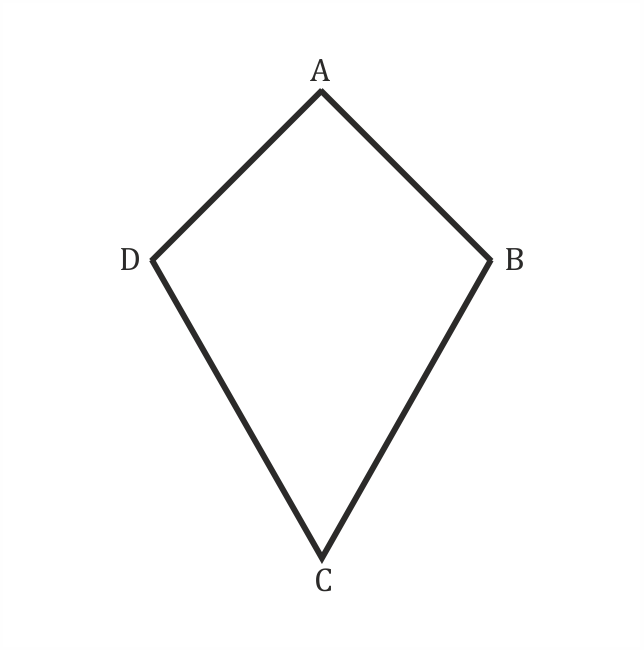30-06-2020    382 times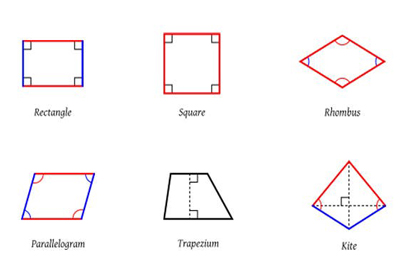We can draw any geometric shape with four sides or edges and four corners or vertices are known as quadrilaterals.

Every quadrilateral has some important properties:

• Every quadrilateral has 4 sides, 4 angles and 4 vertices.
• The sum of its interior angles are 360°.

1. Rectangle: A quadrilateral with equal interior angles i.e. 90° each and opposite sides are equals.2. Squares: A quadrilateral with equal interior angles i.e. 90° each and sides are equals.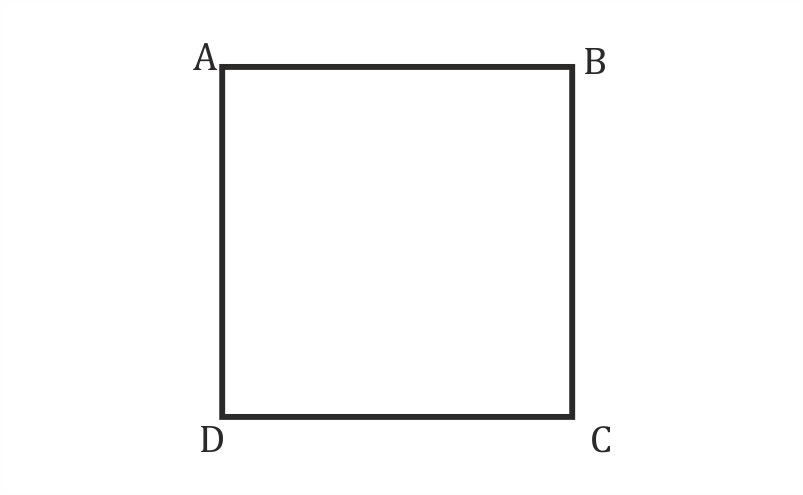3. Trapezium: A quadrilateral with one opposite side is parallel and other opposite sides are non-parallel. But sides are not equals.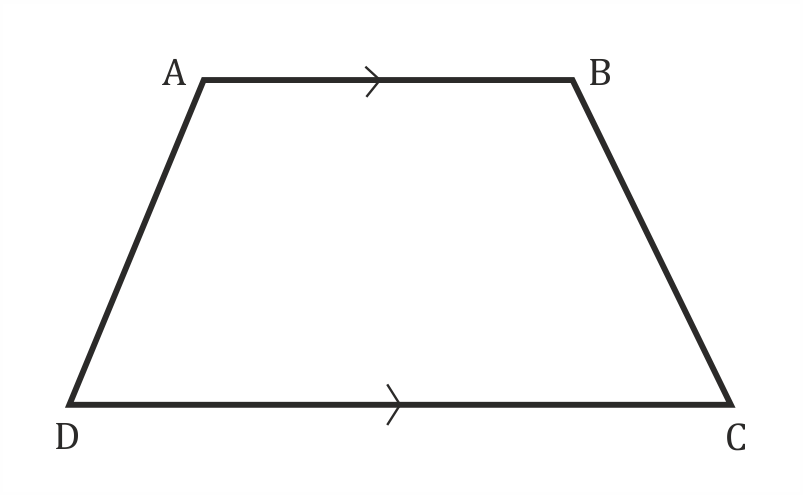4.5. Parallelogram: A quadrilateral with equal and parallel opposite sides. But interior angles are not equal.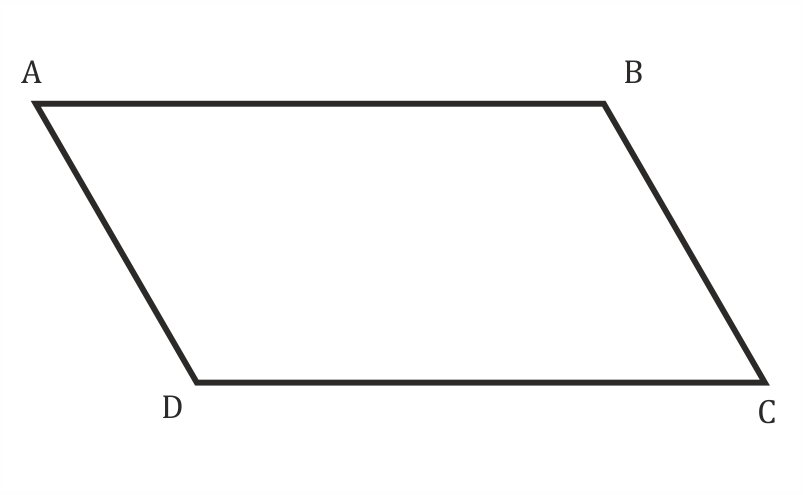6. Rhombus: It is the same as a parallelogram with four sides are equals.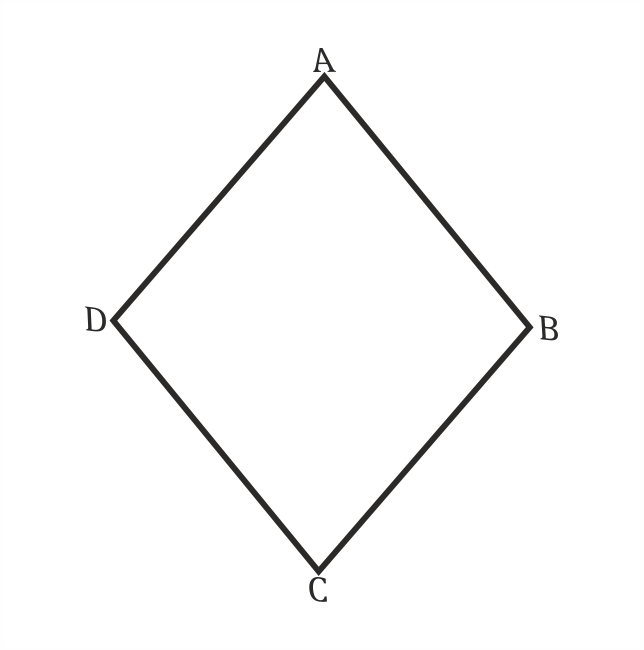7. Kite: If two pair of adjacent sides is equal in a quadrilateral then it is known as Kite.